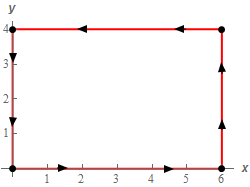Paul's Online Notes
Home / Calculus III / Line Integrals / Green's Theorem
Show Mobile Notice Show All Notes Hide All Notes
Mobile Notice
You appear to be on a device with a "narrow" screen width (i.e. you are probably on a mobile phone). Due to the nature of the mathematics on this site it is best views in landscape mode. If your device is not in landscape mode many of the equations will run off the side of your device (should be able to scroll to see them) and some of the menu items will be cut off due to the narrow screen width.

### Section 5-7 : Green's Theorem

4. Use Green’s Theorem to evaluate $$\displaystyle \int\limits_{C}{{\left( {{y^4} - 2y} \right)\,dx - \left( {6x - 4x{y^3}} \right)\,dy}}$$ where $$C$$ is shown below.Show All Steps Hide All Steps

Start Solution

Okay, first let’s notice that if we walk along the path in the direction indicated then our left hand will be over the enclosed area and so this path does have the positive orientation and we can use Green’s Theorem to evaluate the integral.

From the integral we have,

$P = {y^4} - 2y\hspace{0.25in}\hspace{0.25in}Q = - \left( {6x - 4x{y^3}} \right) = 4x{y^3} - 6x$

Remember that $$P$$ is multiplied by $$x$$ and $$Q$$ is multiplied by $$y$$ and don’t forget to pay attention to signs. It is easy to get in a hurry and miss a sign in front of one of the terms.

Show Step 2

Using Green’s Theorem the line integral becomes,

$\int\limits_{C}{{\left( {{y^4} - 2y} \right)\,dx - \left( {4x{y^3} - 6x} \right)\,dy}} = \iint\limits_{D}{{4{y^3} - 6 - \left( {4{y^3} - 2} \right)\,dA}} = \iint\limits_{D}{{ - 4\,dA}} = - 4\iint\limits_{D}{{\,dA}}$

$$D$$ is the region enclosed by the curve.

Show Step 3

Okay, we are now pretty much done with this problem. After factoring out the “-4” from the integral we can use the following fact to finish the evaluation of the integral.

\begin{align*}\int\limits_{C}{{\left( {{y^4} - 2y} \right)\,dx - \left( {4x{y^3} - 6x} \right)\,dy}} & = - 4\iint\limits_{D}{{\,dA}}\\ & = - 4\left( {{\mbox{Area of }}D} \right) = - 4\left[ {\left( 6 \right)\left( 4 \right)} \right] = \require{bbox} \bbox[2pt,border:1px solid black]{{ - 96}}\end{align*}

Don’t forget the fact that,

$\iint\limits_{D}{{\,dA}} = \mbox{Area of }D$

This is a very useful fact. It doesn’t come up often but when it does it can reduce the amount of work required to finish out a problem.

Of course, this fact is really only useful if $$D$$ is a region that we can easily determine the area for and in this case $$D$$ was just a rectangle that we could easily get the width, 6, and the height, 4, from the graph in the problem statement.